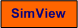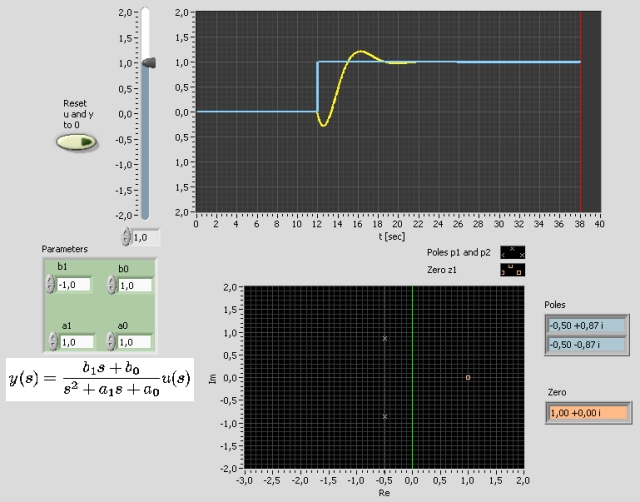# Transfer Function

Snapshot of the front panel of the simulator:## Description of the simulated system

The following general second order transfer function model is simulated:

y(s)/u(s) = H(s) = (b1s + b0)/(s2 +  a1s + a0)

H(s) can have both poles and zeros. You can determine the coefficients of the numerator and the denominator polynomial.

## Aims

The aims of this simulator is to develop the understanding of the relation between poles and zeros and the time response of transfer function models.

## Motivation

Transfer function models are frequently used in signal processing (to represent signal filters) and control  theory (to represent process models, controllers, and sensors).